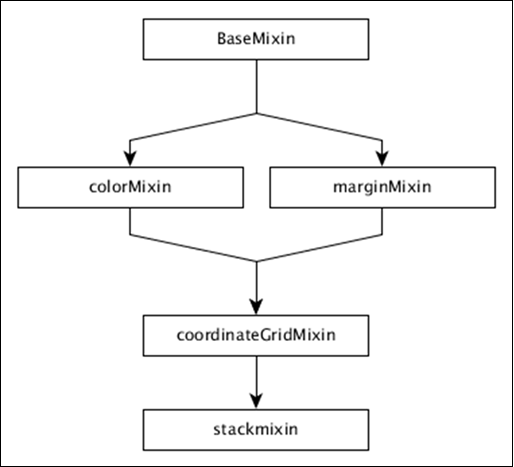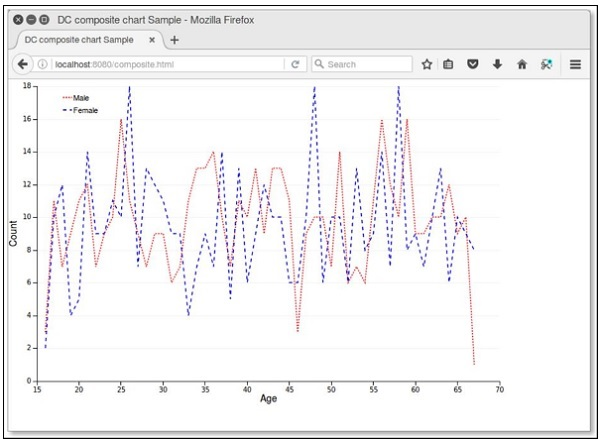# DC.js - Series Chart

A series is a set of data. You can plot a chart based on the data. This chapter explains how to draw a series chart in detail.

## Series Chart Methods

Before moving on to draw a series chart, we should understand the dc.seriesChart class and its methods. The dc.seriesChart uses Mixins to get the basic functionality of drawing a chart. The mixin used by the dc.seriesChart is −

• dc.stackMixin

The complete class diagram of the dc.seriesChart is as follows −The dc.seriesChart gets all the methods of the above specified mixins. It has its own methods to draw the series chart, which are explained below −

### chart( [function])

This method is used to get or set the chart function.

### seriesAccessor( [accessor])

It is used to get or set the accessor function for the displayed series.

### seriesSort( [sortFunction])

This method is used to get or set a function to sort the list of series by giving series values.

### valueSort( [sortFunction])

This method is used to get or set a function to sort the values of each series.

## Draw a Series Chart

Let us draw a series chart in DC. In this example, let us take a dataset named as people_hw.csv. The sample data file is as follows −

```id,name,gender,height,weight
1,Kinsley,Male,168,90
2,Dimitry,Male,177,61
3,Martica,Female,152,76
4,Brittni,Female,156,88
5,Phillip,Male,161,78
6,Sofie,Female,161,71
7,Avril,Female,163,55
8,Allistir,Male,161,75
9,Emelda,Female,154,66
10,Camella,Female,153,52

...............
...............
```

The above sample file contains many records. You can download the file by clicking the following link and save it to your DC location.

people_hw.csv

Now, let us adhere to the following steps to draw a series chart in DC.

### Step 1: Define a variable

Let us define the variable as shown below −

```var chart = dc.seriesChart('#line');
```

Here, the seriesChart function is mapped with the id line.

### Step 2: Read the data

Read data from the people_hw.csv file −

```d3.csv("data/people_hw.csv", function(errors, people) {
var mycrossfilter = crossfilter(people);
}
```

If data is not present, then it returns an error. Now, assign the data to a crossfilter. Once we get the data, we can retrieve it one by one and check the gender using the coding given below −

```people.forEach(function(x) {
if(x.gender == 'Male') {
x.newdata = 1;
} else {
x.newdata = 2;
}
});
```

### Step 3: Create an age dimension

Now, create a dimension for age as shown below −

```var hwDimension = mycrossfilter.dimension(function(data) {
return [data.gender, data.height];
});
```

Here, we have assigned the dimension and it returns the gender and the height. Now, group it using the reduceCount() function, which is defined below −

```var hwGroup = hwDimension.group().reduceCount();
```

### Step 4: Generate a chart

Now, generate a series chart using the coding given below −

```chart
.width(800)
.height(600)
.chart(function(c) {
})

.x(d3.scale.linear().domain([145,180]))
.elasticY(true)
.brushOn(false)
.xAxisLabel("Height")
.yAxisLabel("Count")
.dimension(hwDimension)
.group(hwGroup)
.seriesAccessor(function(d) { return d.key;})
.keyAccessor(function(d) { return +d.key; })
.valueAccessor(function(d) { return +d.value; })
legend(dc.legend().x(350).y(500).itemHeight(13).gap(5).horizontal(1).legendWidth(120)\
.itemWidth(60));

chart.render();
```

Here,

• Chart width is 800 and height is 600.
• Using the method d3.scale.linear(), we specify the domain value.
• Using the seriesAccessor function, it displays the series for the datum.
• Key and value accessor returns the key and value from the series.
• Legend can be used to add height and width.

### Step 5: Working example

The complete code listing is as follows. Create a web page line_series.html and add the following changes in it.

```<html>
<title>Series chart Sample</title>
<link rel = "stylesheet" type = "text/css" href = "css/bootstrap.css">
<link rel = "stylesheet" type = "text/css" href = "css/dc.css"/>

<script src = "js/d3.js"></script>
<script src = "js/crossfilter.js"></script>
<script src = "js/dc.js"></script>

<body>
<div>
<div id = "line"></div>
</div>

<script language = "javascript">
var chart = dc.seriesChart('#line');

d3.csv("data/people_hw.csv", function(errors, people) {
var mycrossfilter = crossfilter(people);

people.forEach(function(x) {
if(x.gender == 'Male') {
x.newdata = 1;
} else {
x.newdata = 2;
}
});

var hwDimension = mycrossfilter.dimension(function(data) {
return [data.gender, data.height];
});
var hwGroup = hwDimension.group().reduceCount();

chart
.width(800)
.height(600)
.chart(function(c) {
})
.x(d3.scale.linear().domain([145,180]))
.elasticY(true)
.brushOn(false)
.xAxisLabel("Height")
.yAxisLabel("Count")
.dimension(hwDimension)
.group(hwGroup)
.seriesAccessor(function(d) { return d.key;})
.keyAccessor(function(d) { return +d.key; })
.valueAccessor(function(d) { return +d.value; })
.legend(dc.legend().x(350).y(500).itemHeight(13).gap(5).horizontal(1)
.legendWidth(120).itemWidth(60));

chart.render();
});
</script>
</body>
</html>
```

Now, request the browser and we will see the following response.